# MSBSHSE Solutions For Class 9 Maths Part 2 Chapter 7 Coordinate Geometry

MSBSHSE Solutions For Class 9 Maths Part 2 Chapter 7 Coordinate Geometry helps students to improve their knowledge in Coordinate Geometry. BYJU’S provides the students with error free solutions, which are prepared by our specialised professionals. These solutions help students not only to strengthen their foundation in the subject, but also to crack different types of problems easily. This chapter deals with axis, origin, quadrant and coordinates of a point in a plane. Students can easily access and download MSBSHSE Solutions For Class 9 Maths Part 2 Chapter 7 Coordinate Geometry from our website in PDF format.

## Download the PDF of Maharashtra Solutions For Class 9 Maths Part 2 Chapter 7 Coordinate Geometry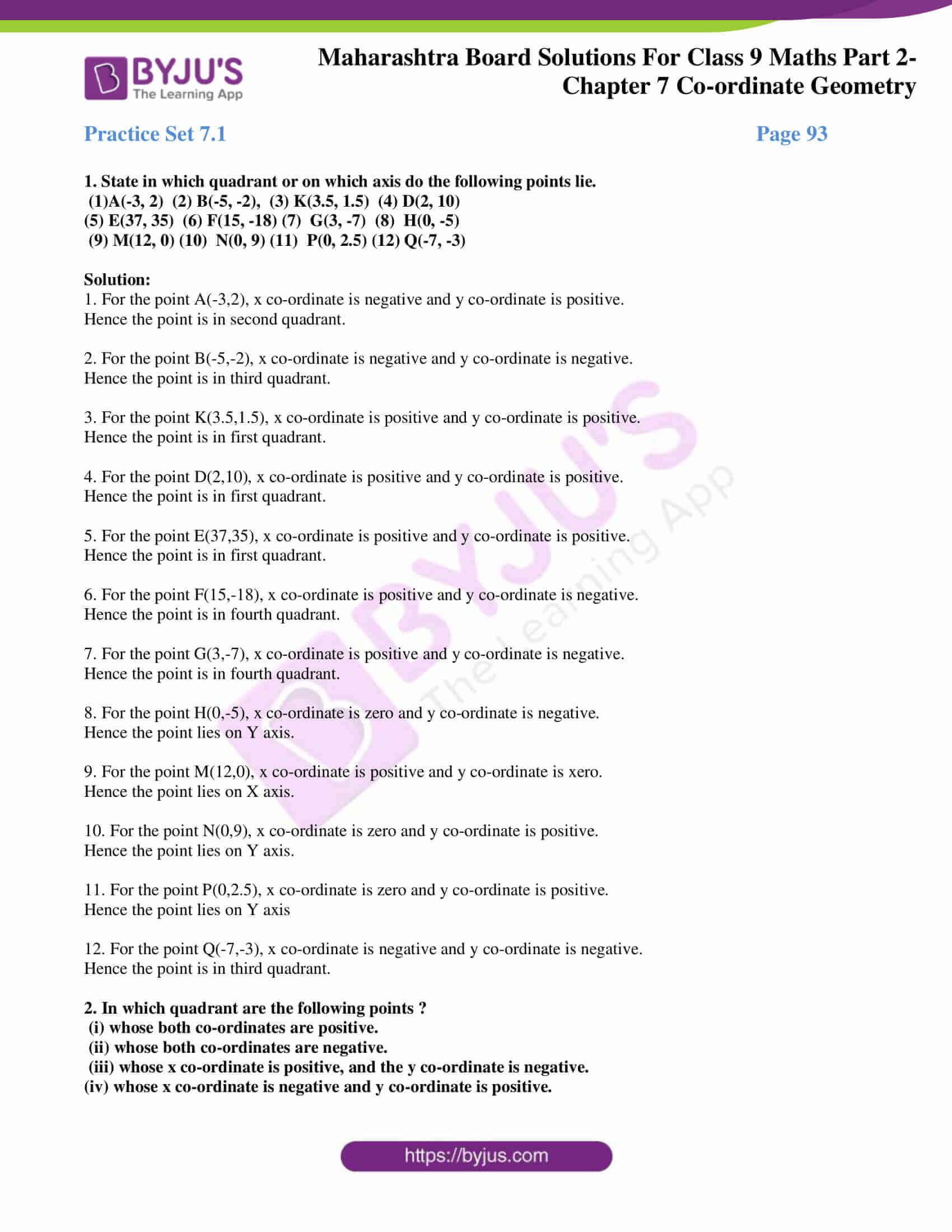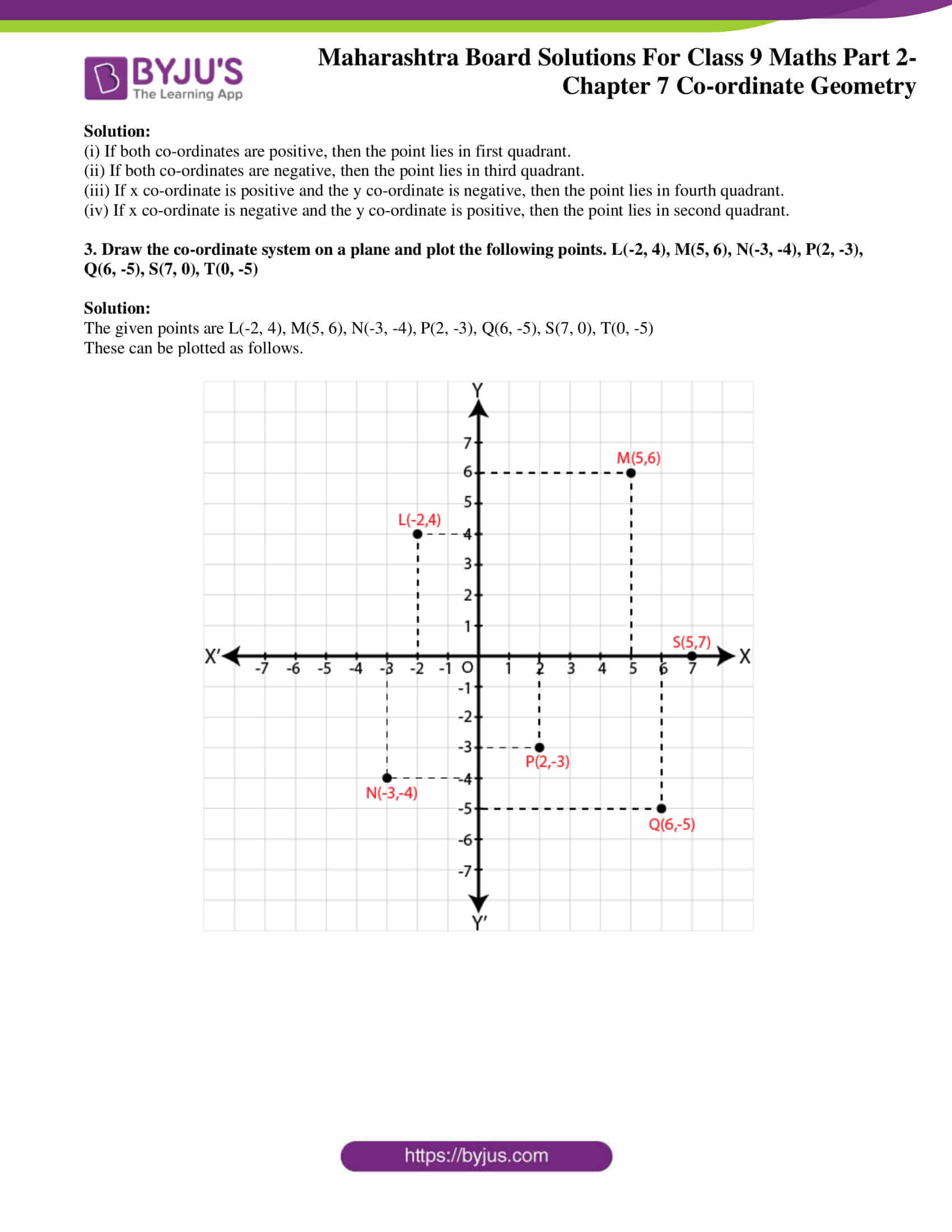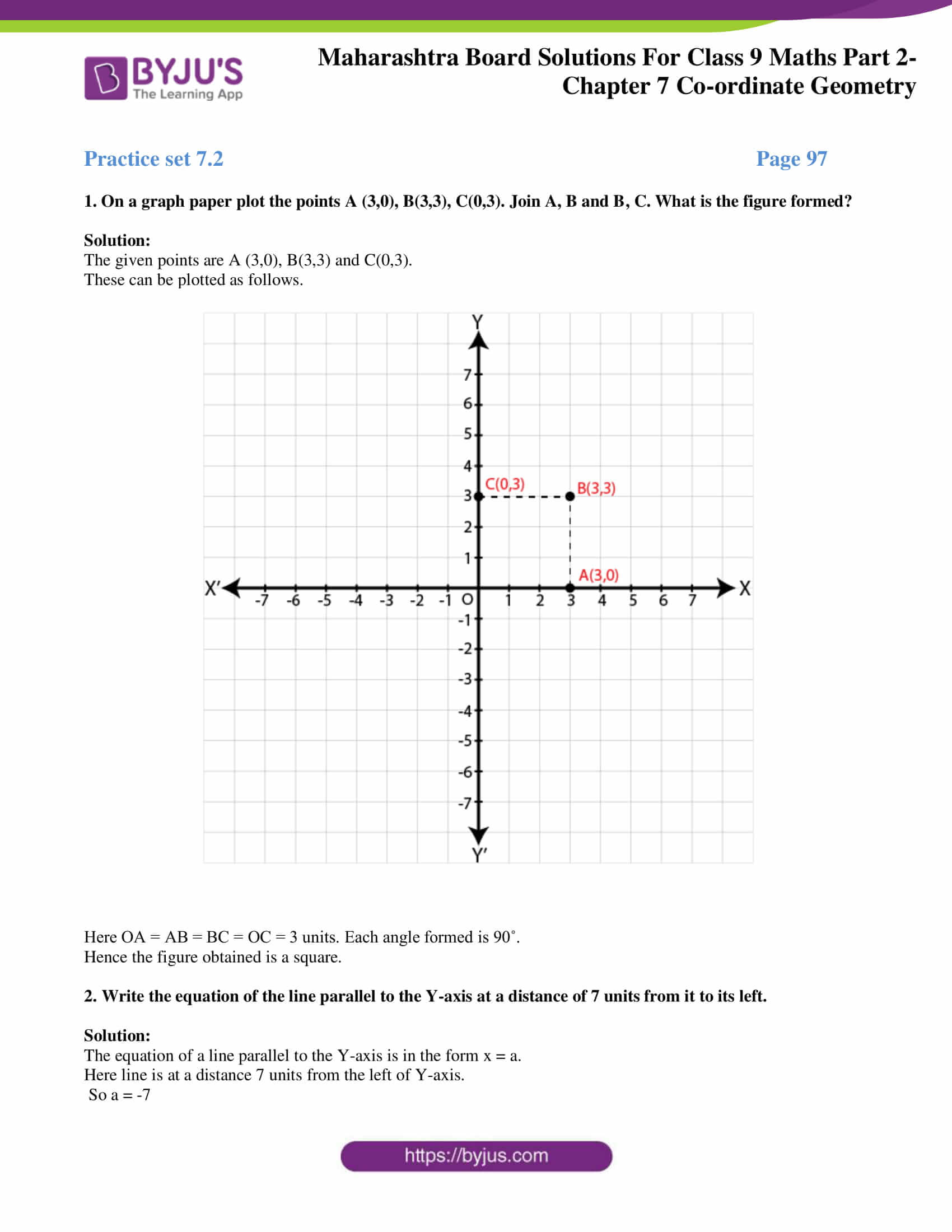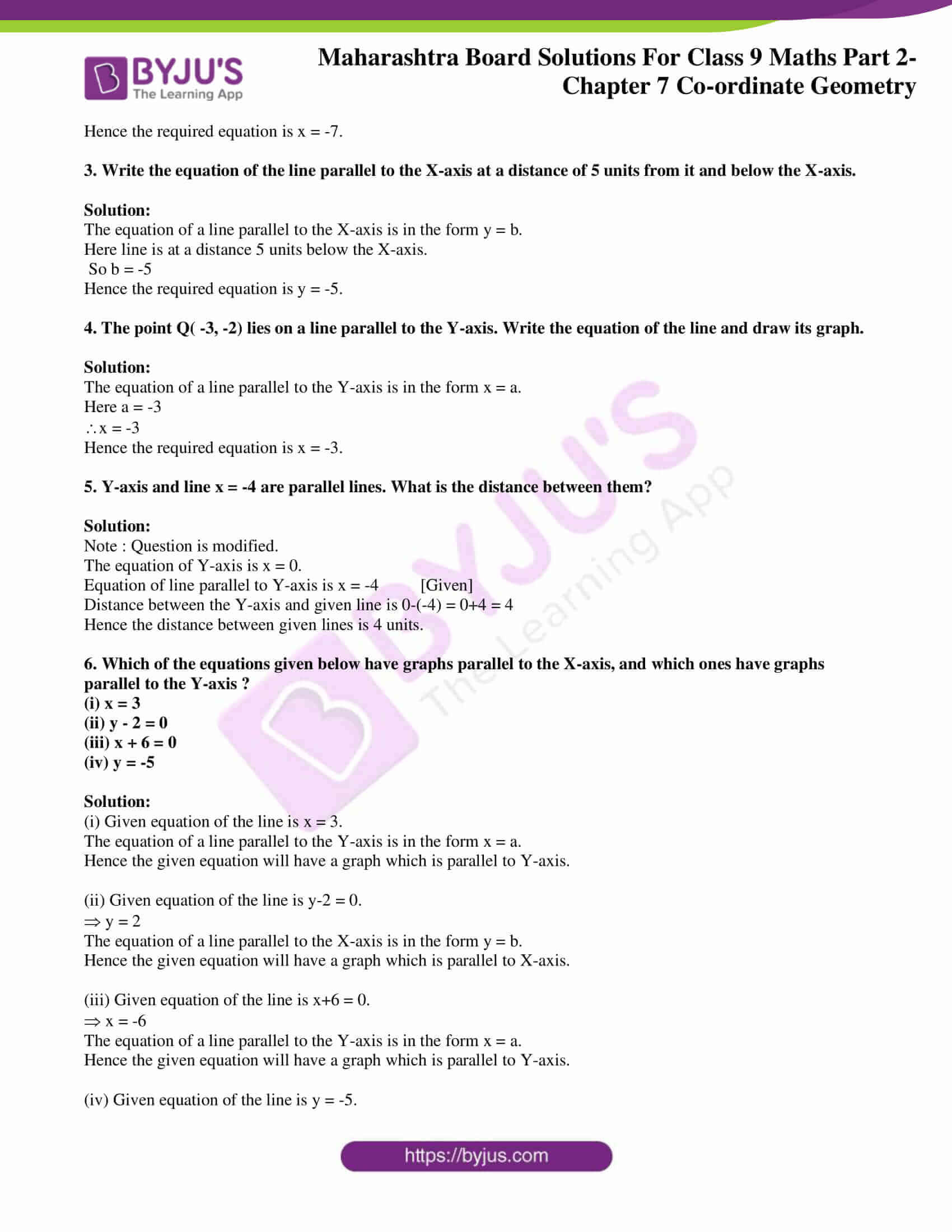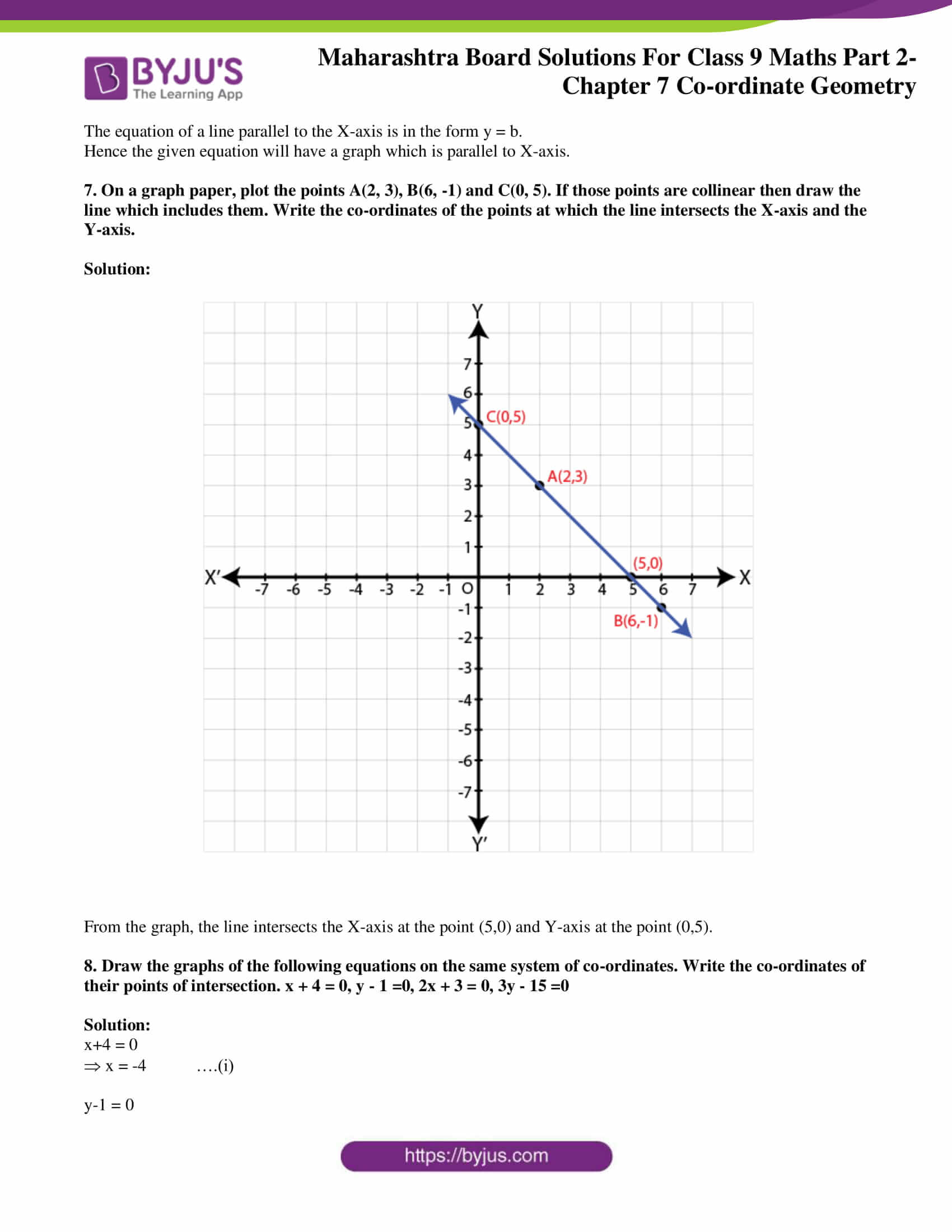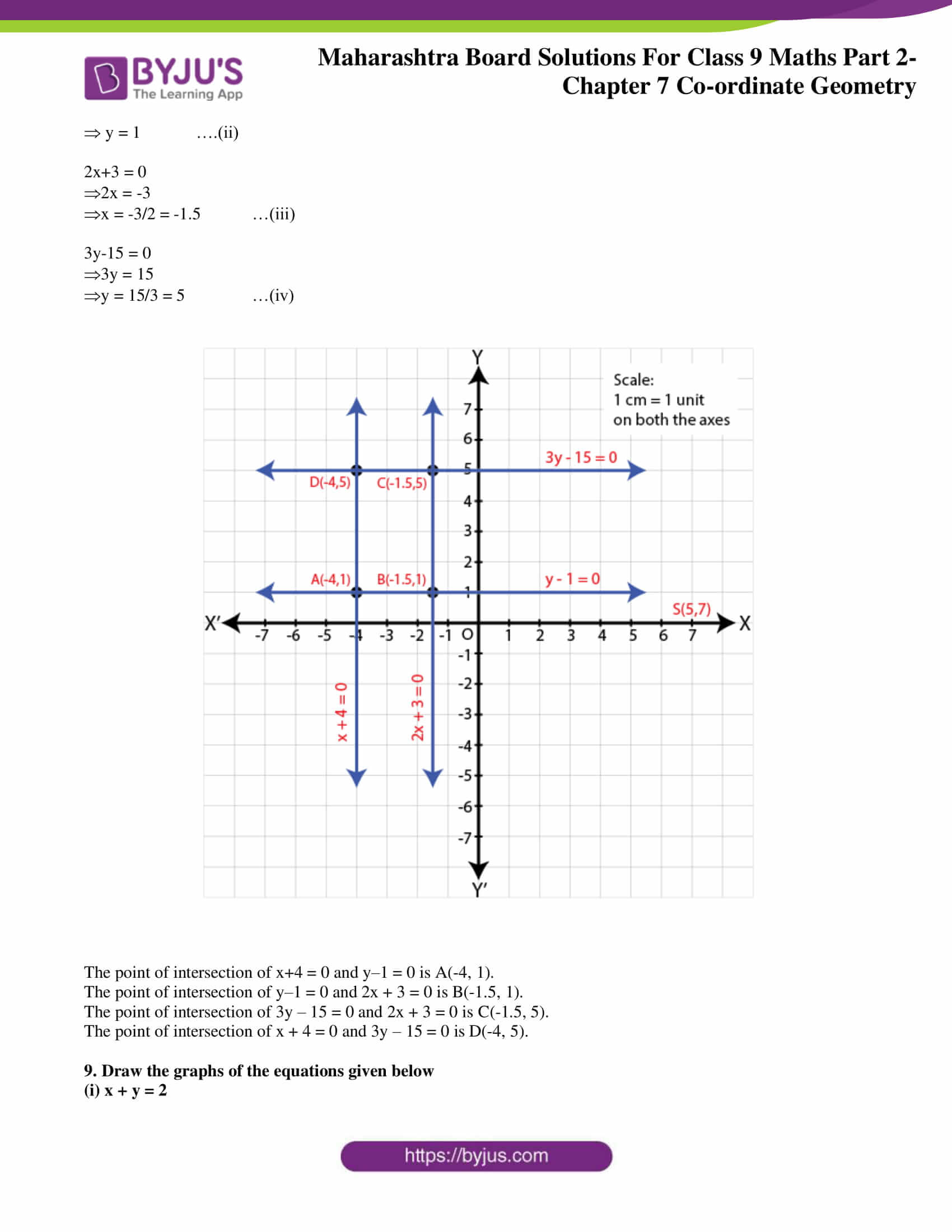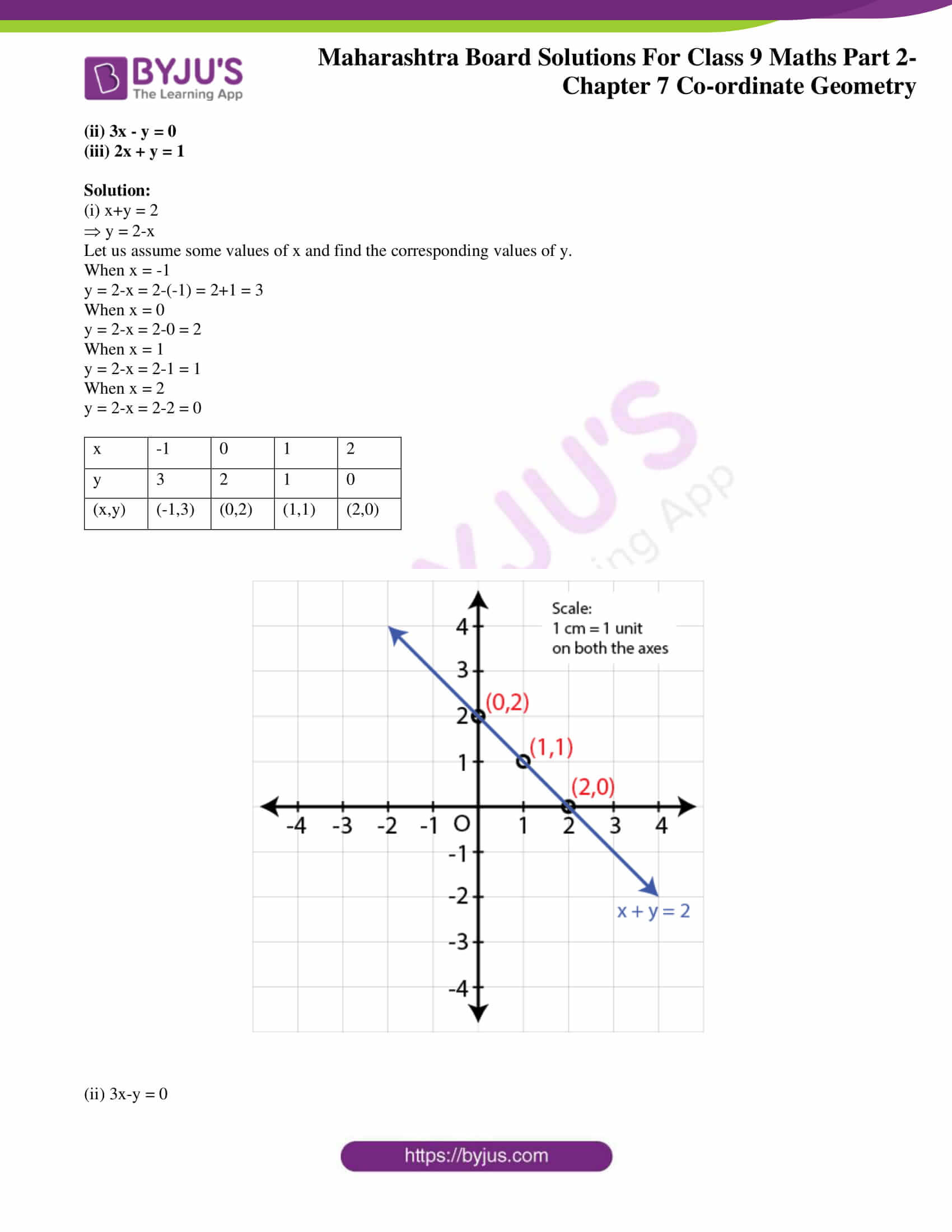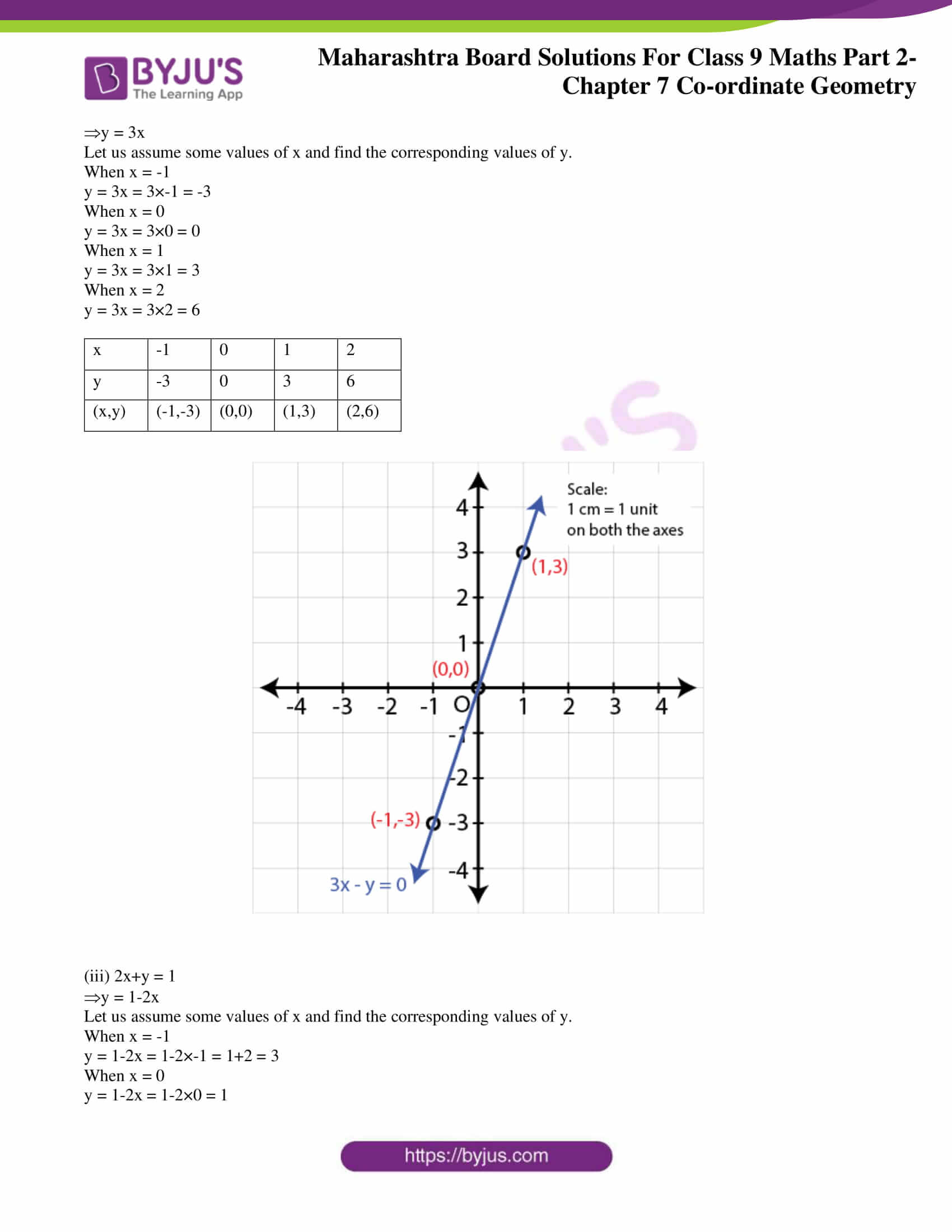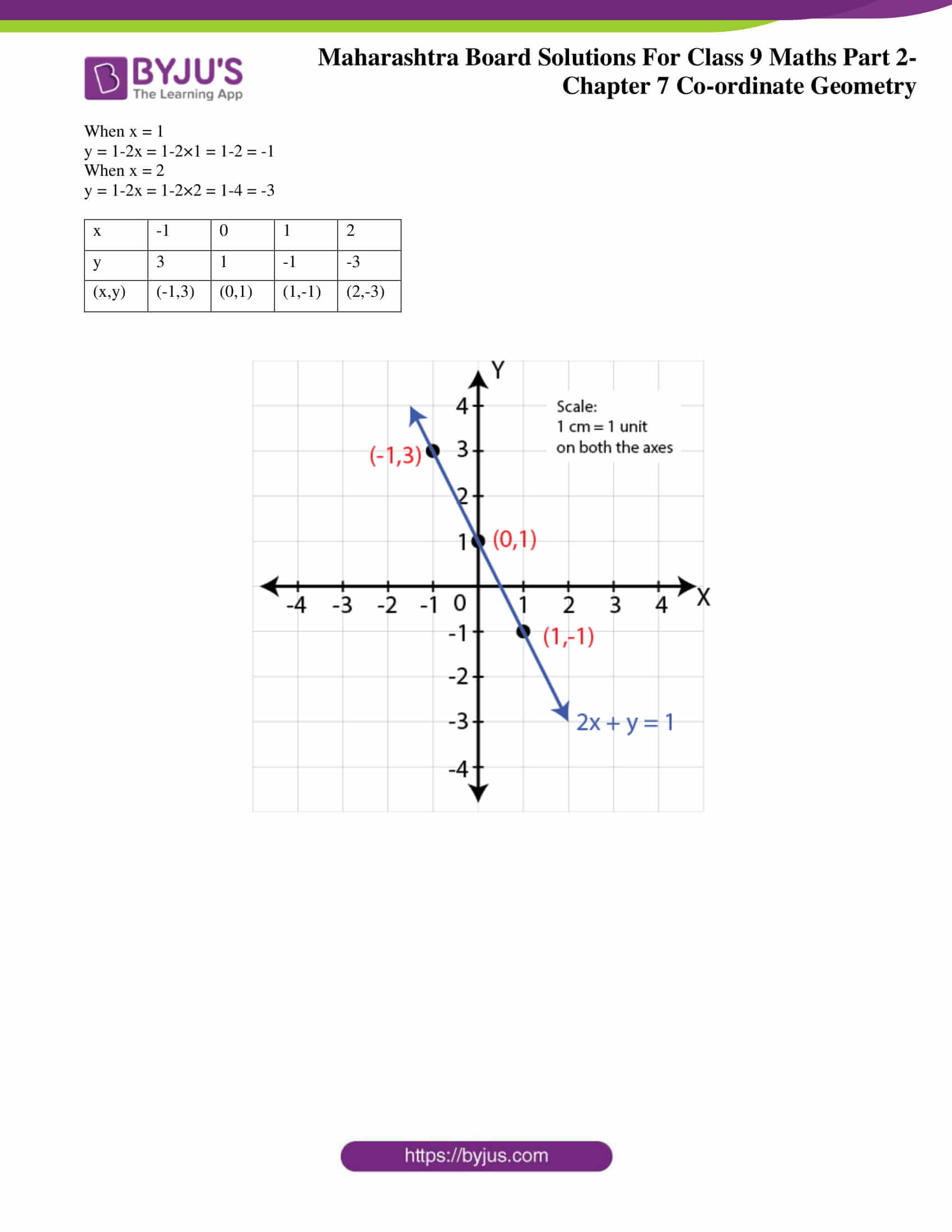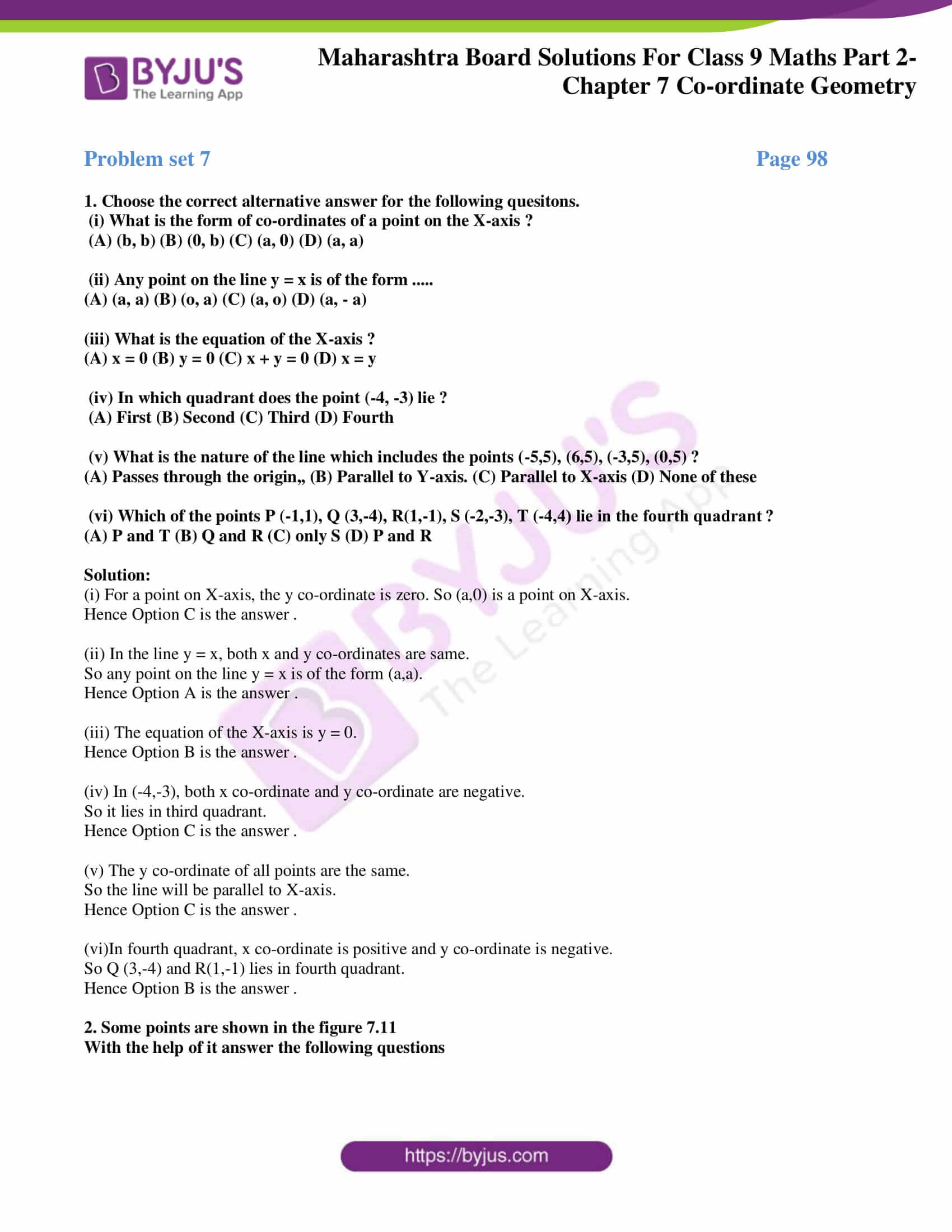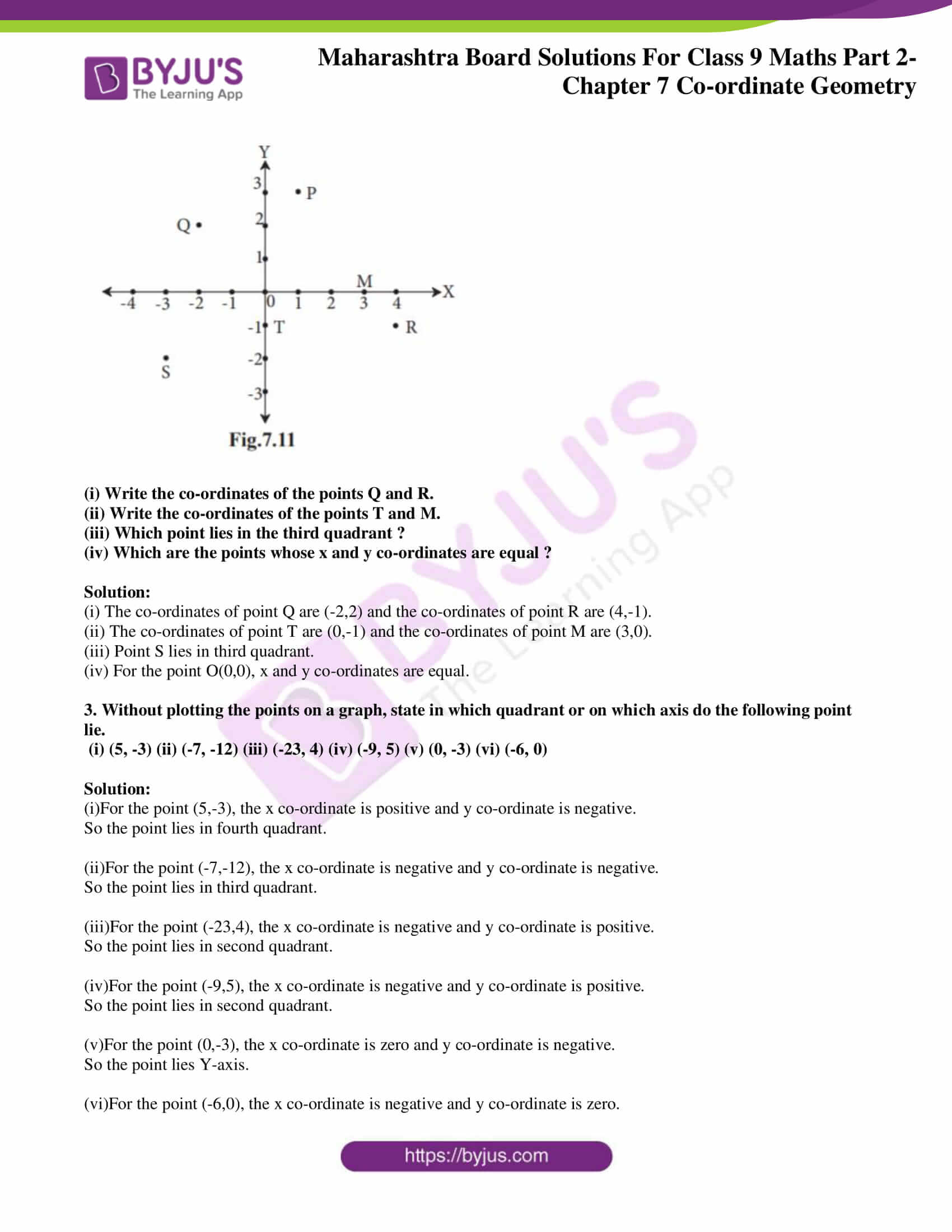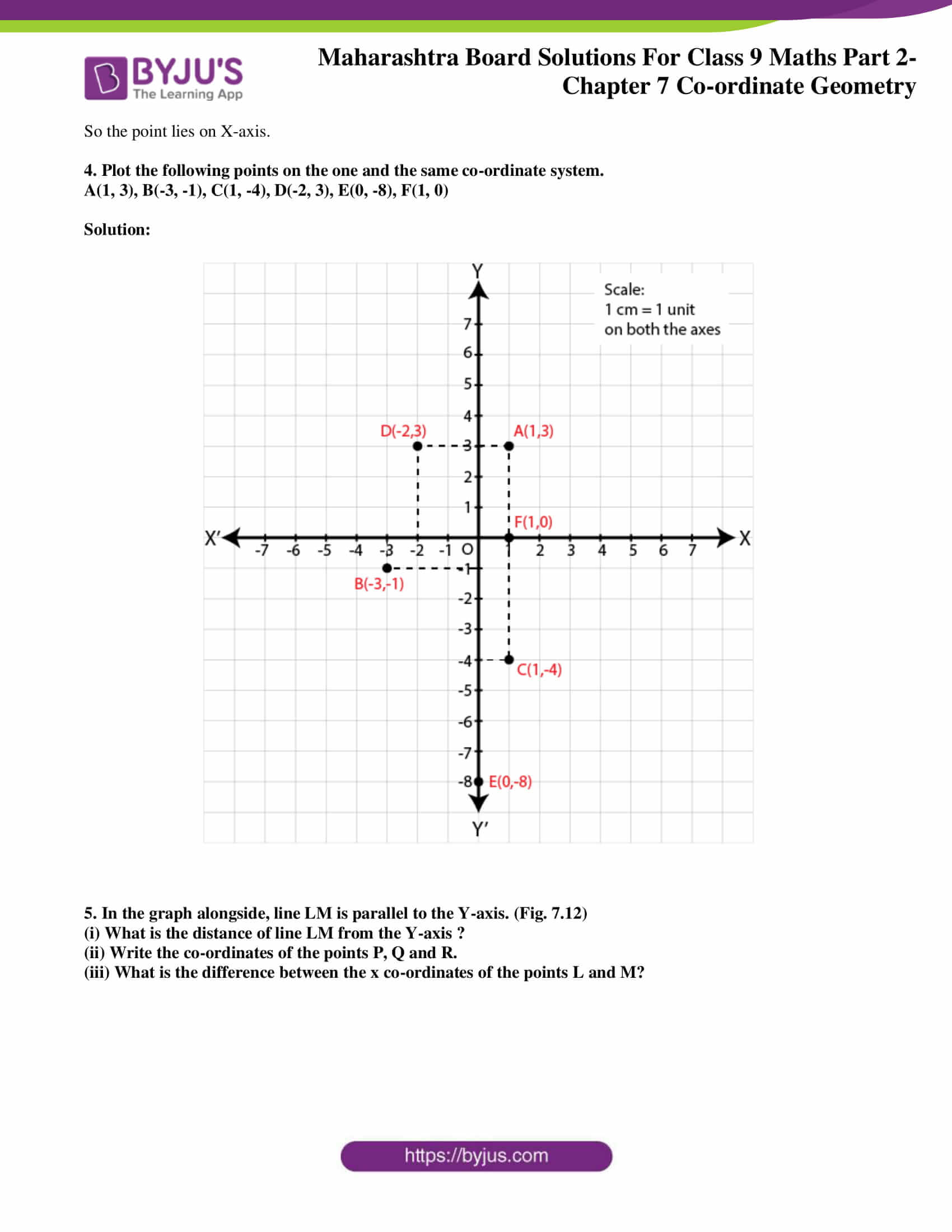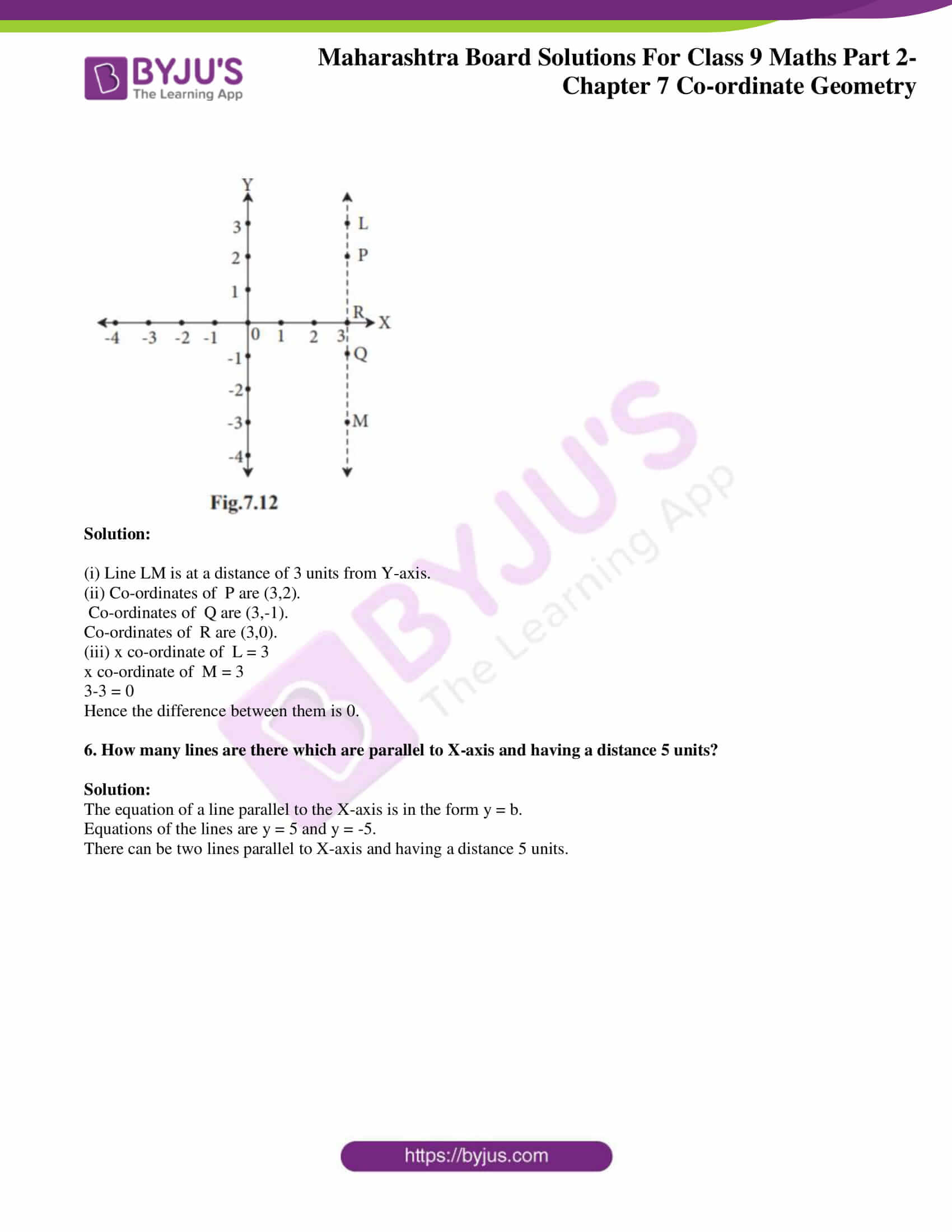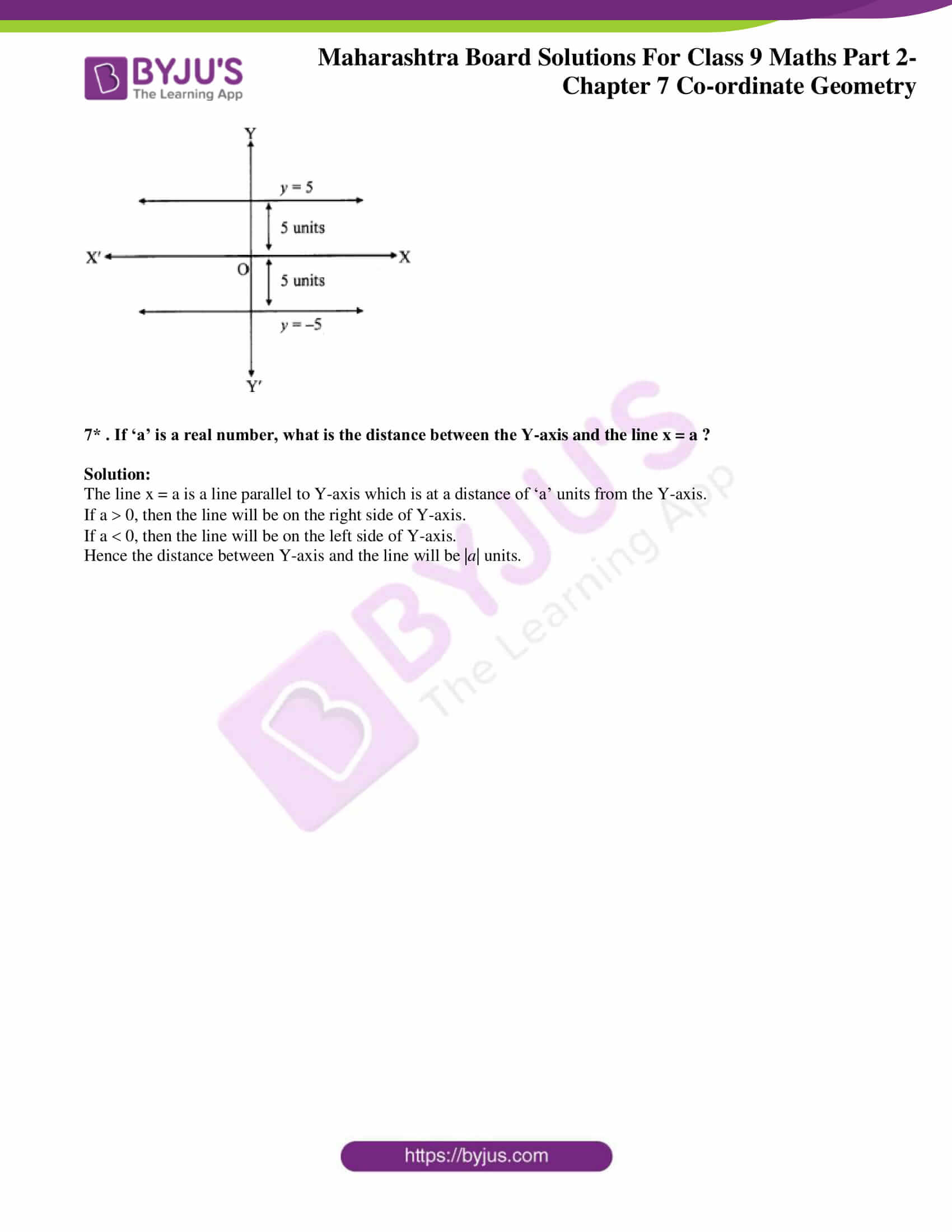### Access answers to Maths MSBSHSE Solutions For Class 9 Part 2 Chapter 7 – Coordinate Geometry

Practice Set 7.1 Page 93

1. State in which quadrant or on which axis do the following points lie.

(1)A(-3, 2) (2) B(-5, -2), (3) K(3.5, 1.5) (4) D(2, 10)

(5) E(37, 35) (6) F(15, -18) (7) G(3, -7) (8) H(0, -5)

(9) M(12, 0) (10) N(0, 9) (11) P(0, 2.5) (12) Q(-7, -3)

Solution:

1. For the point A(-3,2), x co-ordinate is negative and y co-ordinate is positive.

Hence the point is in second quadrant.

2. For the point B(-5,-2), x co-ordinate is negative and y co-ordinate is negative.

Hence the point is in third quadrant.

3. For the point K(3.5,1.5), x co-ordinate is positive and y co-ordinate is positive.

Hence the point is in first quadrant.

4. For the point D(2,10), x co-ordinate is positive and y co-ordinate is positive.

Hence the point is in first quadrant.

5. For the point E(37,35), x co-ordinate is positive and y co-ordinate is positive.

Hence the point is in first quadrant.

6. For the point F(15,-18), x co-ordinate is positive and y co-ordinate is negative.

Hence the point is in fourth quadrant.

7. For the point G(3,-7), x co-ordinate is positive and y co-ordinate is negative.

Hence the point is in fourth quadrant.

8. For the point H(0,-5), x co-ordinate is zero and y co-ordinate is negative.

Hence the point lies on Y axis.

9. For the point M(12,0), x co-ordinate is positive and y co-ordinate is xero.

Hence the point lies on X axis.

10. For the point N(0,9), x co-ordinate is zero and y co-ordinate is positive.

Hence the point lies on Y axis.

11. For the point P(0,2.5), x co-ordinate is zero and y co-ordinate is positive.

Hence the point lies on Y axis

12. For the point Q(-7,-3), x co-ordinate is negative and y co-ordinate is negative.

Hence the point is in third quadrant.

2. In which quadrant are the following points ?

(i) whose both co-ordinates are positive.

(ii) whose both co-ordinates are negative.

(iii) whose x co-ordinate is positive, and the y co-ordinate is negative.

(iv) whose x co-ordinate is negative and y co-ordinate is positive.

Solution:

(i) If both co-ordinates are positive, then the point lies in first quadrant.

(ii) If both co-ordinates are negative, then the point lies in third quadrant.

(iii) If x co-ordinate is positive and the y co-ordinate is negative, then the point lies in fourth quadrant.

(iv) If x co-ordinate is negative and the y co-ordinate is positive, then the point lies in second quadrant.

3. Draw the co-ordinate system on a plane and plot the following points. L(-2, 4), M(5, 6), N(-3, -4), P(2, -3), Q(6, -5), S(7, 0), T(0, -5)

Solution:

The given points are L(-2, 4), M(5, 6), N(-3, -4), P(2, -3), Q(6, -5), S(7, 0), T(0, -5)

These can be plotted as follows.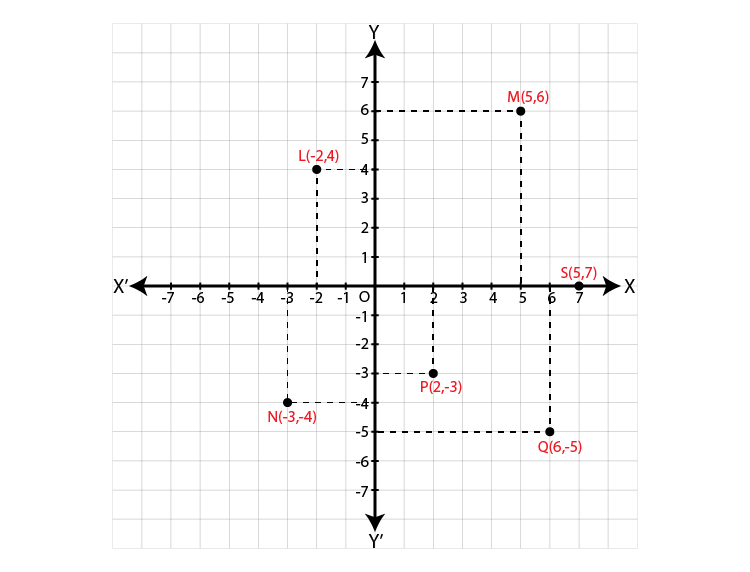Practice set 7.2 Page 97

1. On a graph paper plot the points A (3,0), B(3,3), C(0,3). Join A, B and B, C. What is the figure formed?

Solution:

The given points are A (3,0), B(3,3) and C(0,3).

These can be plotted as follows.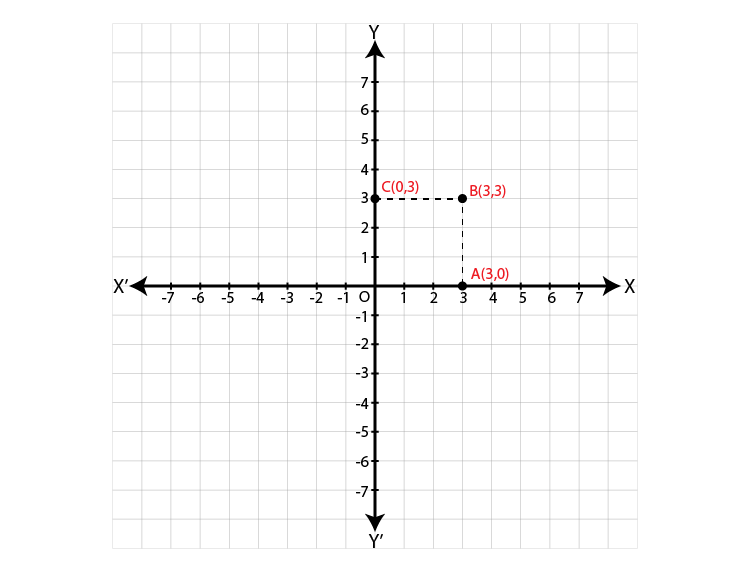Here OA = AB = BC = OC = 3 units. Each angle formed is 90˚.

Hence the figure obtained is a square.

2. Write the equation of the line parallel to the Y-axis at a distance of 7 units from it to its left.

Solution:

The equation of a line parallel to the Y-axis is in the form x = a.

Here line is at a distance 7 units from the left of Y-axis.

So a = -7

Hence the required equation is x = -7.

3. Write the equation of the line parallel to the X-axis at a distance of 5 units from it and below the X-axis.

Solution:

The equation of a line parallel to the X-axis is in the form y = b.

Here line is at a distance 5 units below the X-axis.

So b = -5

Hence the required equation is y = -5.

4. The point Q( -3, -2) lies on a line parallel to the Y-axis. Write the equation of the line and draw its graph.

Solution:

The equation of a line parallel to the Y-axis is in the form x = a.

Here a = -3

x = -3

Hence the required equation is x = -3.

5. Y-axis and line x = -4 are parallel lines. What is the distance between them?

Solution:

Note : Question is modified.

The equation of Y-axis is x = 0.

Equation of line parallel to Y-axis is x = -4 [Given]

Distance between the Y-axis and given line is 0-(-4) = 0+4 = 4

Hence the distance between given lines is 4 units.

6. Which of the equations given below have graphs parallel to the X-axis, and which ones have graphs parallel to the Y-axis ?

(i) x = 3

(ii) y – 2 = 0

(iii) x + 6 = 0

(iv) y = -5

Solution:

(i) Given equation of the line is x = 3.

The equation of a line parallel to the Y-axis is in the form x = a.

Hence the given equation will have a graph which is parallel to Y-axis.

(ii) Given equation of the line is y-2 = 0.

y = 2

The equation of a line parallel to the X-axis is in the form y = b.

Hence the given equation will have a graph which is parallel to X-axis.

(iii) Given equation of the line is x+6 = 0.

x = -6

The equation of a line parallel to the Y-axis is in the form x = a.

Hence the given equation will have a graph which is parallel to Y-axis.

(iv) Given equation of the line is y = -5.

The equation of a line parallel to the X-axis is in the form y = b.

Hence the given equation will have a graph which is parallel to X-axis.

7. On a graph paper, plot the points A(2, 3), B(6, -1) and C(0, 5). If those points are collinear then draw the line which includes them. Write the co-ordinates of the points at which the line intersects the X-axis and the Y-axis.

Solution: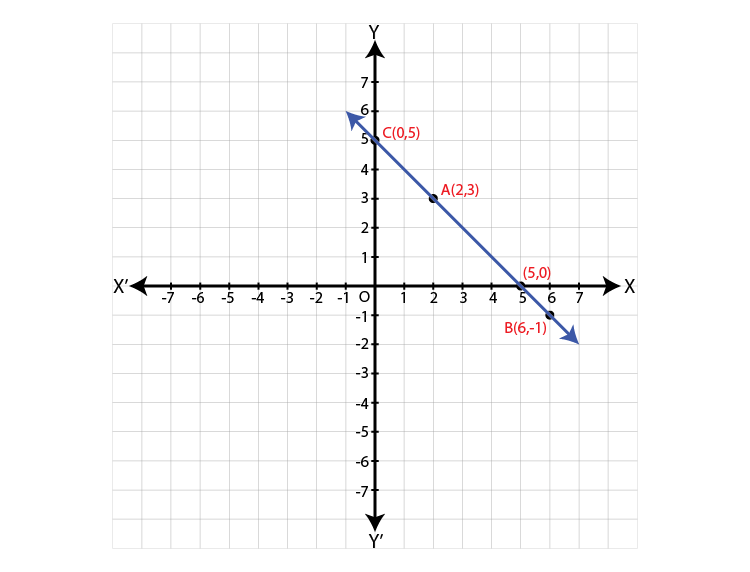From the graph, the line intersects the X-axis at the point (5,0) and Y-axis at the point (0,5).

8. Draw the graphs of the following equations on the same system of co-ordinates. Write the co-ordinates of their points of intersection. x + 4 = 0, y – 1 =0, 2x + 3 = 0, 3y – 15 =0

Solution:

x+4 = 0

x = -4 ….(i)

y-1 = 0

y = 1 ….(ii)

2x+3 = 0

2x = -3

x = -3/2 = -1.5 …(iii)

3y-15 = 0

3y = 15

y = 15/3 = 5 …(iv)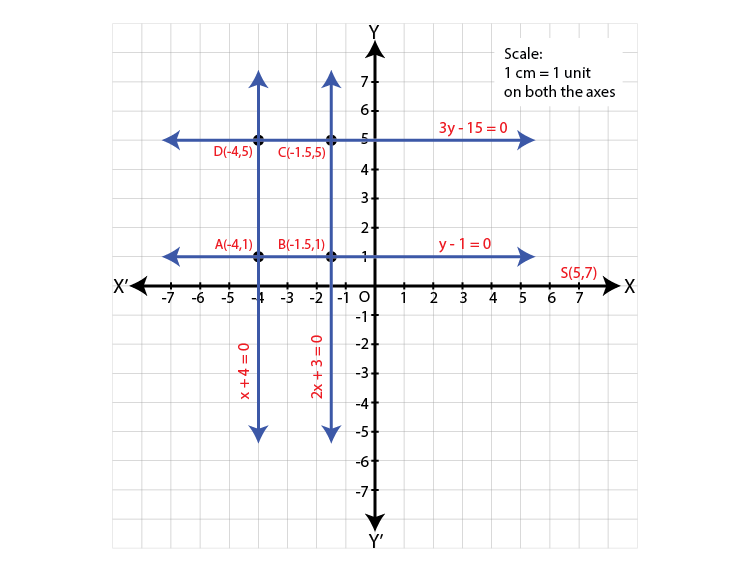The point of intersection of x+4 = 0 and y–1 = 0 is A(-4, 1).

The point of intersection of y–1 = 0 and 2x + 3 = 0 is B(-1.5, 1).

The point of intersection of 3y – 15 = 0 and 2x + 3 = 0 is C(-1.5, 5).

The point of intersection of x + 4 = 0 and 3y – 15 = 0 is D(-4, 5).

9. Draw the graphs of the equations given below

(i) x + y = 2

(ii) 3x – y = 0

(iii) 2x + y = 1

Solution:

(i) x+y = 2

y = 2-x

Let us assume some values of x and find the corresponding values of y.

When x = -1

y = 2-x = 2-(-1) = 2+1 = 3

When x = 0

y = 2-x = 2-0 = 2

When x = 1

y = 2-x = 2-1 = 1

When x = 2

y = 2-x = 2-2 = 0

 x -1 0 1 2 y 3 2 1 0 (x,y) (-1,3) (0,2) (1,1) (2,0)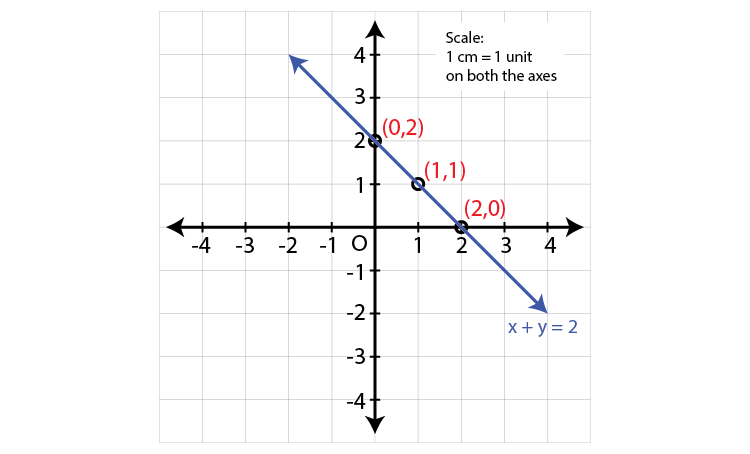(ii) 3x-y = 0

y = 3x

Let us assume some values of x and find the corresponding values of y.

When x = -1

y = 3x = 3×-1 = -3

When x = 0

y = 3x = 3×0 = 0

When x = 1

y = 3x = 3×1 = 3

When x = 2

y = 3x = 3×2 = 6

 x -1 0 1 2 y -3 0 3 6 (x,y) (-1,-3) (0,0) (1,3) (2,6)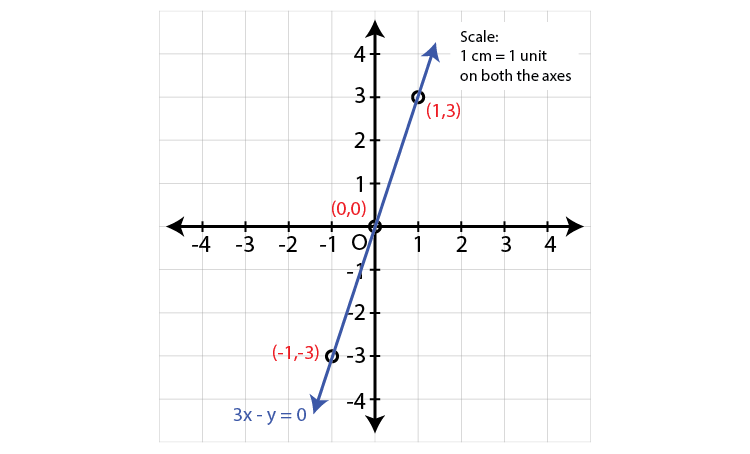(iii) 2x+y = 1

y = 1-2x

Let us assume some values of x and find the corresponding values of y.

When x = -1

y = 1-2x = 1-2×-1 = 1+2 = 3

When x = 0

y = 1-2x = 1-2×0 = 1

When x = 1

y = 1-2x = 1-2×1 = 1-2 = -1

When x = 2

y = 1-2x = 1-2×2 = 1-4 = -3

 x -1 0 1 2 y 3 1 -1 -3 (x,y) (-1,3) (0,1) (1,-1) (2,-3)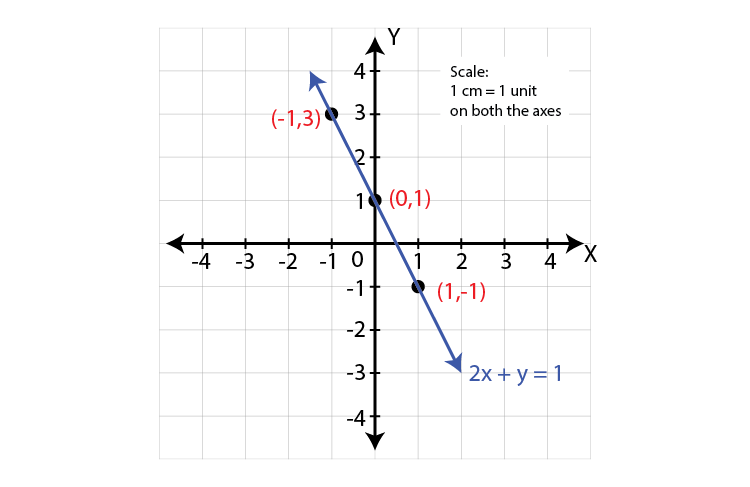Problem set 7 Page 98

1. Choose the correct alternative answer for the following quesitons.

(i) What is the form of co-ordinates of a point on the X-axis ?

(A) (b, b) (B) (0, b) (C) (a, 0) (D) (a, a)

(ii) Any point on the line y = x is of the form …..

(A) (a, a) (B) (o, a) (C) (a, o) (D) (a, – a)

(iii) What is the equation of the X-axis ?

(A) x = 0 (B) y = 0 (C) x + y = 0 (D) x = y

(iv) In which quadrant does the point (-4, -3) lie ?

(A) First (B) Second (C) Third (D) Fourth

(v) What is the nature of the line which includes the points (-5,5), (6,5), (-3,5), (0,5) ?

(A) Passes through the origin,, (B) Parallel to Y-axis. (C) Parallel to X-axis (D) None of these

(vi) Which of the points P (-1,1), Q (3,-4), R(1,-1), S (-2,-3), T (-4,4) lie in the fourth quadrant ?

(A) P and T (B) Q and R (C) only S (D) P and R

Solution:

(i) For a point on X-axis, the y co-ordinate is zero. So (a,0) is a point on X-axis.

Hence Option C is the answer .

(ii) In the line y = x, both x and y co-ordinates are same.

So any point on the line y = x is of the form (a,a).

Hence Option A is the answer .

(iii) The equation of the X-axis is y = 0.

Hence Option B is the answer .

(iv) In (-4,-3), both x co-ordinate and y co-ordinate are negative.

So it lies in third quadrant.

Hence Option C is the answer .

(v) The y co-ordinate of all points are the same.

So the line will be parallel to X-axis.

Hence Option C is the answer .

(vi)In fourth quadrant, x co-ordinate is positive and y co-ordinate is negative.

So Q (3,-4) and R(1,-1) lies in fourth quadrant.

Hence Option B is the answer .

2. Some points are shown in the figure 7.11

With the help of it answer the following questions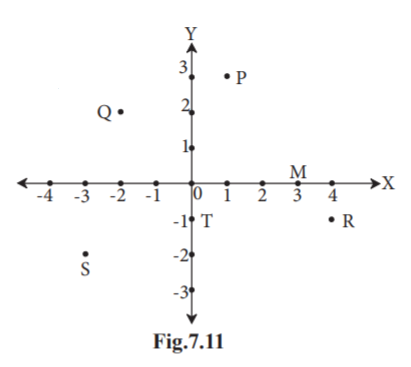(i) Write the co-ordinates of the points Q and R.

(ii) Write the co-ordinates of the points T and M.

(iii) Which point lies in the third quadrant ?

(iv) Which are the points whose x and y co-ordinates are equal ?

Solution:

(i) The co-ordinates of point Q are (-2,2) and the co-ordinates of point R are (4,-1).

(ii) The co-ordinates of point T are (0,-1) and the co-ordinates of point M are (3,0).

(iii) Point S lies in third quadrant.

(iv) For the point O(0,0), x and y co-ordinates are equal.

3. Without plotting the points on a graph, state in which quadrant or on which axis do the following point lie.

(i) (5, -3) (ii) (-7, -12) (iii) (-23, 4) (iv) (-9, 5) (v) (0, -3) (vi) (-6, 0)

Solution:

(i)For the point (5,-3), the x co-ordinate is positive and y co-ordinate is negative.

So the point lies in fourth quadrant.

(ii)For the point (-7,-12), the x co-ordinate is negative and y co-ordinate is negative.

So the point lies in third quadrant.

(iii)For the point (-23,4), the x co-ordinate is negative and y co-ordinate is positive.

So the point lies in second quadrant.

(iv)For the point (-9,5), the x co-ordinate is negative and y co-ordinate is positive.

So the point lies in second quadrant.

(v)For the point (0,-3), the x co-ordinate is zero and y co-ordinate is negative.

So the point lies Y-axis.

(vi)For the point (-6,0), the x co-ordinate is negative and y co-ordinate is zero.

So the point lies on X-axis.

4. Plot the following points on the one and the same co-ordinate system.

A(1, 3), B(-3, -1), C(1, -4), D(-2, 3), E(0, -8), F(1, 0)

Solution: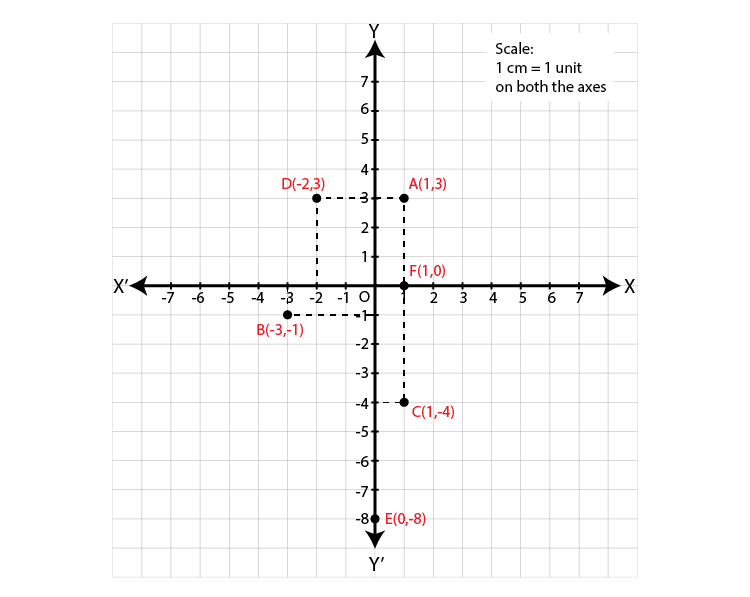5. In the graph alongside, line LM is parallel to the Y-axis. (Fig. 7.12)

(i) What is the distance of line LM from the Y-axis ?

(ii) Write the co-ordinates of the points P, Q and R.

(iii) What is the difference between the x co-ordinates of the points L and M?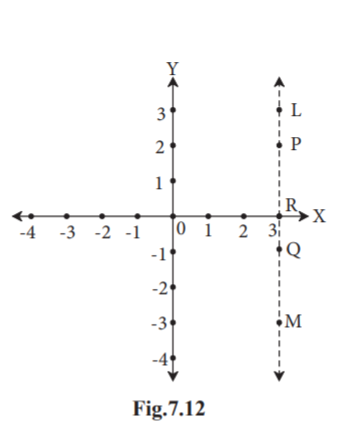Solution:

(i) Line LM is at a distance of 3 units from Y-axis.

(ii) Co-ordinates of P are (3,2).

Co-ordinates of Q are (3,-1).

Co-ordinates of R are (3,0).

(iii) x co-ordinate of L = 3

x co-ordinate of M = 3

3-3 = 0

Hence the difference between them is 0.

6. How many lines are there which are parallel to X-axis and having a distance 5 units?

Solution:

The equation of a line parallel to the X-axis is in the form y = b.

Equations of the lines are y = 5 and y = -5.

There can be two lines parallel to X-axis and having a distance 5 units.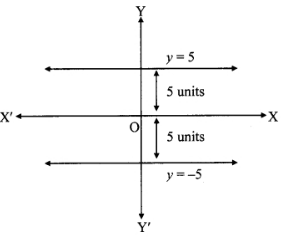7* . If ‘a’ is a real number, what is the distance between the Y-axis and the line x = a ?

Solution:

The line x = a is a line parallel to Y-axis which is at a distance of ‘a’ units from the Y-axis.

If a 0, then the line will be on the right side of Y-axis.

If a 0, then the line will be on the left side of Y-axis.

Hence, the distance between Y-axis and the line will be |a| units.

This chapter, explains the equation of a line, line parallel to X-axis and line parallel to Y-axis. This chapter also describes how to plot a point in a plane. Log on to BYJU’S and enjoy accurate solutions of Coordinate Geometry.

## Frequently Asked Questions on Maharashtra State Board Solutions for Class 9 Maths Part 2 Chapter 7 Coordinate Geometry

### Are these Maharashtra State Board Class 9 Maths Part 2 Chapter 7 Solutions useful to prepare for the exams?

Yes, these Maharashtra State Board Class 9 Maths Part 2 Chapter 7 Solutions can be very helpful. Students are highly recommended to practise these solutions after revising the subjects, as they set the basis for the questions that could get asked often in the board exams. By referring to these solutions after solving the questions will help the students to identify the mistakes and avoid them in the exams.